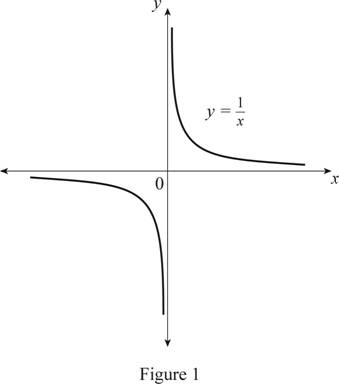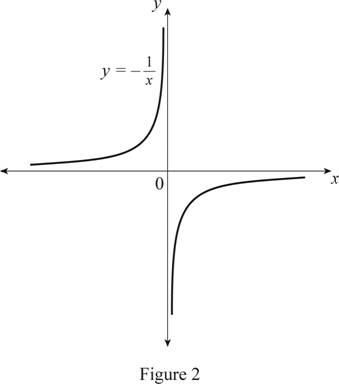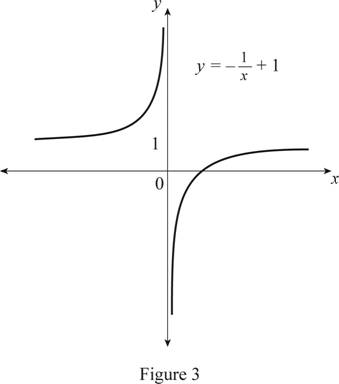# The function y = 1 − 1 x by hand without plotting any points.### Single Variable Calculus: Concepts...

4th Edition
James Stewart
Publisher: Cengage Learning
ISBN: 9781337687805### Single Variable Calculus: Concepts...

4th Edition
James Stewart
Publisher: Cengage Learning
ISBN: 9781337687805

#### Solutions

Chapter 1.3, Problem 12E
To determine

## To sketch: The function y=1−1x by hand without plotting any points.

Expert Solution

### Explanation of Solution

The standard graph of the function y=1x is roughly drawn as shown below in Figure 1.Draw the graph of y=1x, by reflecting the graph y=1x about the x-axis.

Thus, the graph of y=1x is shown below in Figure 2.Observe that Figure 2 is reflecting Figure 1 about the origin.

To draw the graph of y=11x, shift the graph y=1x by 1 unit upward.

Thus, the graph of y=11x is shown below in Figure 3.From Figure 3, it is observed that the graph of the function y=11x never intersects the x-axis.

### Have a homework question?

Subscribe to bartleby learn! Ask subject matter experts 30 homework questions each month. Plus, you’ll have access to millions of step-by-step textbook answers!